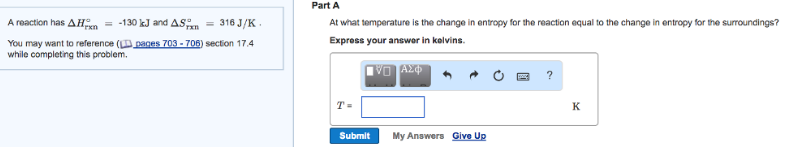Chemistry Practice Problems Entropy Practice Problems Solution: A reaction had ΔH°rxn = - 130 kJ and ΔS°rxn = 316 ...

🤓 Based on our data, we think this question is relevant for Professor Matejovic's class at Ryerson University.

# Solution: A reaction had ΔH°rxn = - 130 kJ and ΔS°rxn = 316 J/K. Part AAt what temperature is the change in entropy for the reaction equal to the change in entropy for the surroundings? Express your answer in kelvins.

###### Problem

A reaction had ΔH°rxn = - 130 kJ and ΔS°rxn = 316 J/K.

Part A

At what temperature is the change in entropy for the reaction equal to the change in entropy for the surroundings? Express your answer in kelvins.View Complete Written Solution

Entropy

Entropy

#### Q. Calculate the standard entropy change for the following reaction at 25 °C. S° values can be found here. HCl (g) + NaOH (s) → NaCl (s) + H2O (l)

Solved • Wed Aug 08 2018 14:18:37 GMT-0400 (EDT)

Entropy

#### Q. Use the data from this table of thermodynamic properties to calculate the values of delta S°rxn for each of the following reactions at 25 °C. (a) 4NH3...

Solved • Wed Aug 08 2018 10:51:25 GMT-0400 (EDT)

Entropy

#### Q. Calculate the standard entropy change for the following reaction at 25 °C. S° values can be found here.C3H8 (g) + 5O2 (g) → 3CO2 (g) + 4H2O (g)

Solved • Tue Aug 07 2018 17:47:27 GMT-0400 (EDT)

Entropy

#### Q. Identify the process in which the entropy increases.a. the phase transition from a solid to a gasb. the phase transition from a gas to a solidc. the p...

Solved • Mon Jul 30 2018 17:01:31 GMT-0400 (EDT)